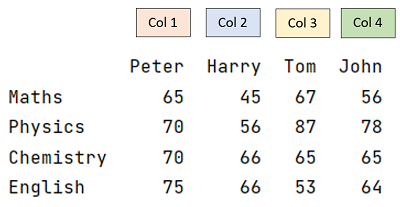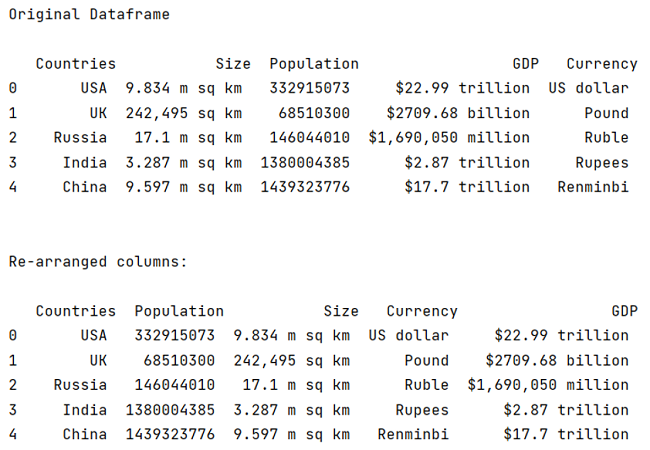# How to change the order of DataFrame columns?

In this tutorial, we will learn how can we change the order of columns in a Panda DataFrame? By Pranit Sharma Last updated : April 10, 2023

## What is order of columns in a Panda DataFrame?

The order of columns refers to the horizontal sequence in which the column names are created.In the figure, the order of columns is Peter -> Harry -> Tom -> Jhon.

Sometimes we might need to rearrange this sequence. Pandas allow us to rearrange the order of columns using the loc Property.

## How to change the order of columns in a Panda DataFrame?

The loc[] property is usually used for selecting rows and columns with the help of their names, but when it comes to rearrange the order of columns, loc[] property is beneficial in more than one way. We can rearrange the order of all the columns or we can rearrange those columns that we want. It takes two arguments, the number of rows and the number of columns.

Defining a colon (:) means selecting all the rows followed by a comma and a list of rearranged column names is the actual syntax to accomplish the task.

## Syntax

```df.loc[:,['col_name','col_name','col_name','col_name']]
```

## Python example to change the order of DataFrame columns

```# Importing Pandas library
import pandas as pd

# Creating a dictionary
d = {
'Countries':['USA','UK','Russia','India','China'],
'Size':['9.834 m sq km','242,495 sq km','17.1 m sq km','3.287 m sq km',
'9.597 m sq km'],
'Population':[332915073,68510300,146044010,1380004385,1439323776],
'GDP':['\$22.99 trillion','\$2709.68 billion',
'\$1,690,050 million','\$2.87 trillion','\$17.7 trillion'],
'Currency':['US  dollar','Pound','Ruble','Rupees','Renminbi']
}

# Creating DataFrame
df = pd.DataFrame(d)

# Printing dataframe
print("\n")
print("Original Dataframe\n",df,"\n")

# Current order of columns is
# Countries -> Size -> Population -> GDP -> Currency
# Using loc method for re-arranging the columns,
# we will store it in new variable
new_df = df.loc[:,['Countries','Population','Size','Currency','GDP']]

# Printing dataframe with re-arranged columns
print("Re-arranged columns:\n",new_df)
```

### Output### Explanation

In the above example, we have passed a list of column names with the new sequence. An important point is that we will pass the list after defining the number of rows. Here, we have selected all the rows.